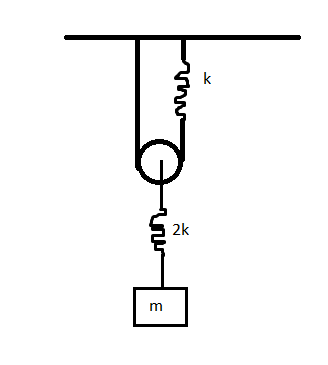# Just hanging around- part 1If the time period of simple harmonic motion of the rectangular block can be represented as $T=2\pi \sqrt{\frac{am}{bk}}$ for coprime positive integers $a,b$.

Find $\sqrt{a^2+b^2}$ up to two decimal places.

Details and Assumptions

• Pulley is smooth and massless, and the strings are light and inextensible.
• Gravity is present.
• The crooked lines in the figure represents springs of spring constant $k$ and $2k$ as shown.

-Take the amplitude of oscillations to be very small.

×